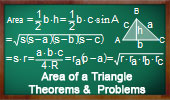# Plane Geometry: Area of a triangle, Theorems and Problems - Table of Content - Page 3Proposed Problem 252.
Rectangle, isosceles right triangle, square, area.

Proposed Problem 248.
Napoleon's Theorem III. Inner and outer Napoleon triangles areas.

Proposed Problem 225. Viviani's Theorem Extension, Regular Polygon, Apothem, Distance. Area of triangles.

Proposed Problem 224. Viviani's theorem, Isosceles triangle, Altitude, Distances, Point on the extension of the base. Area of triangles.

Proposed Problem 223. Viviani's theorem, Isosceles triangle, Altitude, Distances. Area of triangles.

Proposed Problem 222. Viviani's theorem, Equilateral triangle, Exterior point, Distances. Area of triangles.

Proposed Problem 221. Viviani's theorem, Equilateral triangle, Interior point, Distances. Area of triangles.

Lune of Hippocrates Index. Area of a right triangle.

Proposed Problem 212. 120 Degree Triangle, Equilateral triangles, Areas.

Proposed Problem 211. 60 Degree Triangle, Equilateral triangles, Areas.

Problem 206. Area of a Right Triangle, Inradius, and Exradius relative to the hypotenuse.

Proposed Problem 205. Right Triangle Area, Exradii relatives to legs or catheti.

Proposed Problem 197. Area of a Triangle, Side, Inradius, and Exradius.

Proposed Problem 196. Area of triangles, Inradius and Exradii Formula.

Proposed Problem 195. Area of a Triangle, Inradius, Exradii.

Proposed Problem 194. Area of a Triangle, Semiperimeter, Exradius.

Proposed Problem 193. Area of a Triangle, Semiperimeter, Inradius.

Proposed Problem 192. Circle, Diameter, Chord, Perpendicular, Triangle, Area.

Proposed Problem 191. Triangle, Altitudes, Orthocenter, Squares, Areas.

Go to Page: Previous | 1 | 2 | 3 | 4 | 5 | 6 | 7 | 8 | Next

 Home | Geometry | Areas | Email | Post a comment | By Antonio Gutierrez